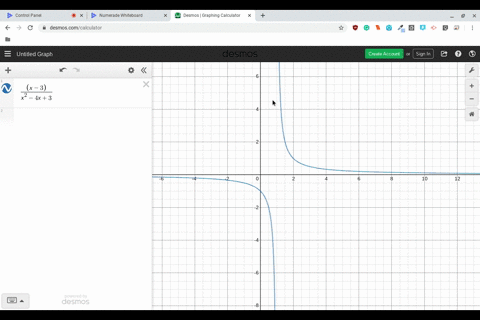Enroll in one of our FREE online STEM bootcamps. Join today and start acing your classes!View Bootcamps### Writing In Exercises 59 and $60,$ use a graphing …

02:07Westview High School
Problem 59

# Writing In Exercises 59 and $60,$ use a graphing utility to graph the function and estimate the limit (if it exists). What is the domain of the function? Can you detect a possible error in determining the domain of a function solely by analyzing the graph generated by a graphing utility? Write a short paragraphabout the importance of examining a function analytically as well as graphically.$$f(x)=\frac{\sqrt{x+5}-3}{x-4}$$$$\lim _{x \rightarrow 4} f(x)$$

## Discussion

You must be signed in to discuss.

## Video Transcript

you're given the falling function f of X equal to skirt if exposed by my Serie over its demise for and you are ask to figure out by graphing dysfunction what the limit as X approaches for is equal to. So if we look at the graph, we can zoom enough for to see what value it seems to be approaching so we can see out for it is undefined, but around it it seems approaching 0.167 So we know that the approximate limit or if you vex approximately 0.167 now next year ossified the domain raised off the graph so we can zoom out a little bit. We can see. Oh, the graph seemed to start a negative five. So her domain is negative five and then it seems to approach overlooking and a graph. The graph seem soo approach. If we zoom out seems to go on and on and on, so seems approaching infinity. We also know that there's a whole Act four because it's undefined. So we can say that the domain is neighbor 5 to 4 and for to infinity now you Now you are then asked to explain why you have to figure this out analytically and not just so like algebra, and not just to really grafts. Well, you know that this is because you don't know if there's any other holes in the graphs you don't. You won't be able to see him. You don't know if the graph cuts off and even a cube zooming out. You don't know if it ends, you know, like past amount that you zoom out. So because of this, you have to you have to, um, so you need to You need to analyze a function analytically, because that way you won't miss any holes holes, and, you know, if the Garth cuts off